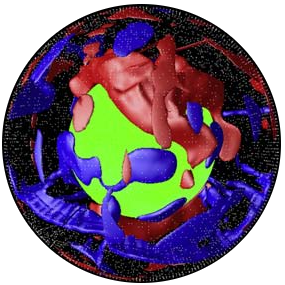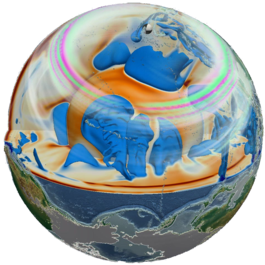A Computational Framework for Mineralogical Thermodynamics of the Earth's Mantle

Thomas C. Chust
<thomas.chust@uni-bayreuth.de>

Material PropertiesTemperature field of a convection simulation from Nakagawa et al. 

\begin{align*} \nabla\cdot \left(\color{red}{\rho} u\right) &= 0, \\ -\nabla p + \color{red}{\eta} \Delta u + \color{red}{\rho} g &= 0, \\ \color{red}{\rho} T \, \left(\frac{\partial}{\partial {t}} + u\cdot\nabla\right) \color{red}{S} &= \color{red}{k} \, \Delta T + \color{red}{\bar\tau} \, \nabla u + \color{red}{\rho H^*}. \end{align*}Global seismic wave propagation simulation from Schuberth et al. 

$$\partial_t^2 x = \color{red}{v}^2 \Delta x, \\ \color{red}{v_p} = \sqrt{\frac{\color{red}{\kappa_S} + \frac43\color{red}{\mu}}{\color{red}{\rho}}}, \color{red}{v_s} = \sqrt{\frac{\color{red}{\mu}}{\color{red}{\rho}}}.$$

Thermodynamic State

Category Properties Dependencies
Thermal $S, C_p$ $\leftarrow \partial_T^{1,2} G$
Elastic $V, \rho, \kappa_S, \color{#88f}{\mu}$ $\leftarrow \partial_{p,T}^{1,2} G$
Transport $k, \eta$ Not linked to $G$
Potentials $E, A, H, G$ $\mathrm{d}G = V\,\mathrm{d}p - S\,\mathrm{d}T$

Birch-Murnaghan–Mie-Grüneisen-Debye ModelProgramming InterfaceProgramming InterfaceProgramming InterfaceProgramming InterfaceTransitions of Perovskite EndmembersEnergetic equilibrium between the $\ce{Mg}$- (blue), $\ce{Fe}$- (red) and $\ce{Al}$-endmembers (green) of bridgmanite and post-perovskite in $p, T$ space.

Transitions of Perovskite EndmembersEnergetic equilibrium between the $\ce{Mg}$- (blue), $\ce{Fe}$- (red) and $\ce{Al}$-endmembers (green) of bridgmanite and post-perovskite in $p, T$ space.

Chemical Equilibrium

In order to compute chemical equilibrium between multiple phases with varying composition, we need three more things:

1. The Gibbs energy of phases modelled as solid solutions has to be computed.
2. The Gibbs energy of aggregates of phases has to be computed.
3. An energetically optimal collection of phases has to be chosen at given $p, T$ and bulk composition.

FMS SystemPhase diagram computed for $\ce{FeO-MgO-SiO2}$ composition with $\frac{\ce{Fe}}{\ce{Fe + Mg}} \approx 0.11$ as a function of $p$ and $T$.

FMS SystemPhase diagram computed for $\ce{FeO-MgO-SiO2}$ composition with $\frac{\ce{Fe}}{\ce{Fe + Mg}} \approx 0.11$ as a function of $p$ and $T$.

FMS SystemPhase diagram computed for $\ce{FeO-MgO-SiO2}$ composition with $\frac{\ce{Fe}}{\ce{Fe + Mg}} \approx 0.11$ as a function of $p$ and $T$.

With the addition of more chemical components,

1. new phases and their stability fields occur,
2. new endmembers are introduced and form mineral solutions whose stability fields generally expand, and/or
3. the variance of mineral assemblages increases, resulting in binary or higher-order phase loops which allow the coexistence of phases previously separated by sharp boundaries.

New PhasesRelative atomic amounts of stable phases for $\ce{FeO-MgO-SiO2}$ composition along the isentrope with a potential temperature of $1600\,\mathrm{K}$.

New PhasesRelative atomic amounts of stable phases for $\ce{CaO-FeO-MgO-SiO2}$ composition along the isentrope with a potential temperature of $1600\,\mathrm{K}$.

Stability FieldsRelative atomic amounts of stable phases for $\ce{FeO-MgO-SiO2}$ composition along the isentrope with a potential temperature of $1600\,\mathrm{K}$.

Stability FieldsRelative atomic amounts of stable phases for $\ce{FeO-MgO-Al2O3-SiO2}$ composition along the isentrope with a potential temperature of $1600\,\mathrm{K}$.

Coexistence RegionsPhase diagram for $\ce{Na2O-CaO-FeO-MgO-Al2O3-SiO2}$ composition as a function of $p$ and $T$. Lines indicate phase boundaries for $\ce{MgSiO3}$ (blue), $\ce{FeSiO3}$ (red) and $\ce{Al2O3}$ endmembers (green) of bridgmanite and post-perovskite.$$\partial_pT\mid_S = \frac{\alpha V}{C_p} T$$

Self-consistently computed isentropes for two different pyrolite-based compositions in comparison with the adiabat from Katsura et al. .

Conclusions

• High accuracy of thermo-elastic parameters or good $p, T$ extrapolation behaviour is possible.
• Calibration of phase equilibria may be specific to certain composition ranges.
• Complex phase transitions buffer latent heat effects and result in smoother adiabats.
• Elastic property profiles computed for homogeneous chemical composition or mechanical mixtures are very similar.

MMA-EoS

https://bitbucket.org/chust/eos
• Versatile simulation software for minerals and mantle aggregates.
• Computes thermoelastic properties and chemical equilibrium states.
• Supports multiple widely used equations of state.
• Features an easy-to-use solution model.
• Offers a free, open and extensible implementation.